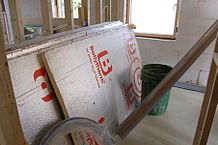# U-valueFigure 1. Certain materials will have a higher R-value, thus corresponding to a lower U-value. Polyisocyanurate insulation is one material with a relatively low U-value.

The U-value of insulation is a value that is used to measure how well a specific type of insulation can resist heat flow. The lower the U-value, the more effective the material is at preventing heat transfer. The U-value is very closely linked to the R-value, as it simply represents its inverse. The U-value is also closely connected to the idea of thermal conductivity, which is a property of a material that determines how well it conducts heat. Windows, doors, and skylights all have a specific U-value associated with how well they insulate.

U-values are used more frequently to determine the insulation properties of windows specifically. A U-value is typically a low number as it represents how much heat energy is lost or gained by the window, and thus a low U-value would be more desirable. As well, U-values are typically used in systems where multiple materials are used, such as in windows whereas R-values are used to determine the insulating capabilities of only one material. In regions where homes need more heating than cooling, it's clear that low U-factors are important, but they are also beneficial in regions where homes need to be cooled. Regardless of the hot or cold, windows with better insulating properties can resist heat flow more efficiently.

## Calculating U-value

When calculating U-value, it is important to know the thickness and area of each building material used so that the total U-value of an assembly can be accurately determined.

Mathematically, U-value is calculated by measuring heat flow across a surface where each side is at a particular temperature. If the R-value is known, simply calculate the inverse of the R-value to obtain the U-value. However, if it is not known the U-value can be calculated as:

• is the temperature difference inside and outside the house in °F
• is the area of the wall (or ceiling) that's being insulated in ft 2
• is how long the measurement took place in seconds
• is how much heat is lost through the wall or window in BTU

## For Further Reading

For further information please see the related pages below:

## Authors and Editors

Bethel Afework, Jordan Hanania, Kailyn Stenhouse, Jason Donev
Last updated: May 18, 2018
Get Citation